﻿ 青春回合 全职业概念站-《新水浒Q传》官方网站

青春回合 全职业概念站

神医

• 阴阳互济心为药
• 亦毒亦医渡苍生
• 使用神奇的葫芦，
• 运用各种增益法术给战斗中的
• 队友以最大的辅助。
• 他们还能够运用神奇的法术复活
• 战斗中牺牲的队友。
•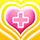甘露术

•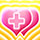天降甘霖

•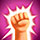聚力诀

•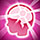归心诀

•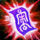咒术反弹

•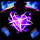清心诀

•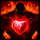医者仁心

•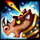疾病传播

•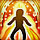危难之护

•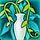慈航普渡

•强化金针

剑圣

• 英气纵横三万里
• 一剑光寒十九洲
• 拥有强健的身体和坚忍的耐力，
• “不求天下无敌，但求无人能欺”
• 是他们毕生的宗旨。
• 进能攻退能守，
• 场上强大的攻防全能手。
•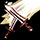截剑式

•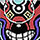袭心诀

•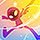虚空闪

•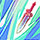七星落长空

•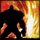以逸待劳

•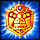金刚护体

•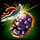声东击西

•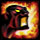火力吸引

•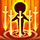复仇

•横扫千军

•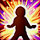星神庇护

武神

• 丧魄灵指逆天宇
• 流星铁拳震穹扉
• 个性不羁好打抱不平，
• 拥有超强的攻击力和敏捷，
• 出拳以快、狠、准著称。
• 优势在于出拳的命中率高，
• 有一击毙命的强大威力
•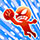爆击

•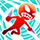闭气指

•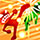反击

•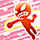破天

•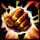破甲一击

•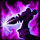暗杀

•隔山打牛

•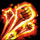连击强化

•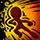冲锋陷阵

•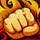拳法风暴

•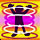意志坚定

箭神

• 策马弯弓辞旧月
• 神箭轻拨定乾坤
• 与生俱来的对自然的感知能力，
• 可在复杂环境中布置陷阱，
• 精通灵兽习性，
• 还可以神箭直中对手要害。
• 拥有不俗的输出、控制能力。
•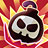地雷陷阱

•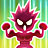巨兽之力

•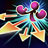乱射

•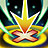致命狙击

•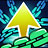束缚射击

•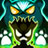灵魂联结

•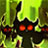适者生存

•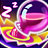睡眠陷阱

•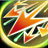箭矢风暴

•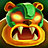野蛮压制

•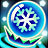霜冻陷阱

法王

• 四象灵妙乘风起
• 法中洪荒未可知
• 神圣而高贵的职业，
• 拥有着最高魔法伤害能力，
• 具备了呼风唤雨的能耐，
• 能够充分利用大自然的灵气
• 作为自己攻击的手段。
•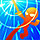寒冰封结

•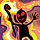苍煌爆炎

•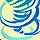风耀神刃

•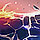大地之怒

•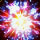魔力炸弹

•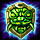魔力盾牌

•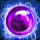法术反射

•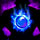魔力引导

•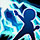法力极效

•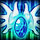心神专注

•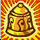金刚罩

道祖

• 银铃一枚 荡平天下
• 清风一缕 吹尽凡尘心
• 天资聪颖，掌握奇能异技
• 使他们能够使用许多玄门法术。
• 这些玄术能够让对手陷入
• 各种异常状态，
• 最大限度地消减对手的能力。
•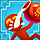醉酒咒

•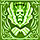影毒降

•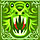恶鬼降

•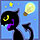咒法领悟

•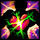石化咒

•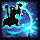法力吸收

•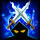灵魂标记

•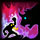驱兽傀儡

•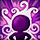气定神闲

•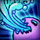法力消耗

•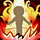仙道之佑

游仙

• 长笛一声惊天际
• 清音几曲入云天
• 灵活多变、八面玲珑的群体，
• 拥有着召唤蕴含灵力的
• “音符”来进行战斗的能力。
• 其手中所持的匕首是沟通、
• 控制“音符”之灵的法器。
•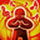凝气

•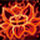红莲诀

•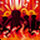裂杀诀

•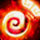炽魂诀

•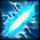裂空诀

•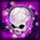魔音咒

•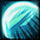玄音斩

•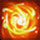株连咒

•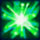如意诀

•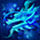水龙吟

•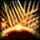绝尘音

• 神医

• 剑圣

• 武神

• 箭神

• 法王

• 道祖

• 游仙